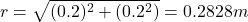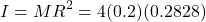How Cheenta works to ensure student success?
Explore the Back-Story

# Moment of InertiaFour small spheres, each of which you can regard as a point of mass (0.2)Kg are arranged in a square (0.4)m on a side and connected by extremely light rods. Find the moment of inertia of the system about an axis through the centre of the square.
Discussion:

The length of each side of a square is (0.4)m. Thus, the perpendicular bisector is at (0.2)m. The length of the bisector dropped from the point of intersection of the diagonals is (0.2)m. Therefore, the distance of the vertices from the point of intersection of diagonals isThe moment of inertia=Four small spheres, each of which you can regard as a point of mass (0.2)Kg are arranged in a square (0.4)m on a side and connected by extremely light rods. Find the moment of inertia of the system about an axis through the centre of the square.
Discussion:

The length of each side of a square is (0.4)m. Thus, the perpendicular bisector is at (0.2)m. The length of the bisector dropped from the point of intersection of the diagonals is (0.2)m. Therefore, the distance of the vertices from the point of intersection of diagonals isThe moment of inertia=This site uses Akismet to reduce spam. Learn how your comment data is processed.

### Knowledge Partner